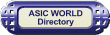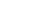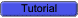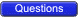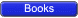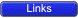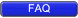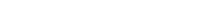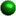Multiplexer

A multiplexer (MUX) is a digital switch which connects data from one of n sources to the output. A number of select inputs determine which data source is connected to the output. The block diagram of MUX with n data sources of b bits wide and s bits wide select line is shown in below figure.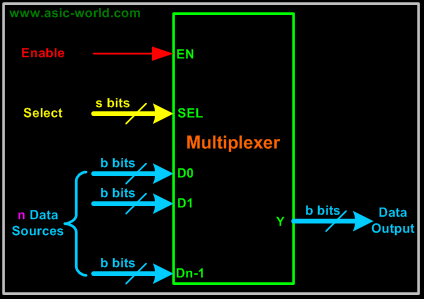MUX acts like a digitally controlled multi-position switch where the binary code applied to the select inputs controls the input source that will be switched on to the output as shown in the figure below. At any given point of time only one input gets selected and is connected to output, based on the select input signal.Mechanical Equivalent of a Multiplexer

The operation of a multiplexer can be better explained using a mechanical switch as shown in the figure below. This rotary switch can touch any of the inputs, which is connected to the output. As you can see at any given point of time only one input gets transferred to output.Example - 2x1 MUX

A 2 to 1 line multiplexer is shown in figure below, each 2 input lines A to B is applied to one input of an AND gate. Selection lines S are decoded to select a particular AND gate. The truth table for the 2:1 mux is given in the table below.Symbol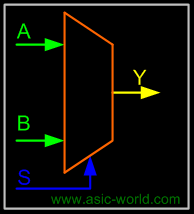Truth Table

 S Y 0 A 1 BDesign of a 2:1 Mux

To derive the gate level implementation of 2:1 mux we need to have truth table as shown in figure. And once we have the truth table, we can draw the K-map as shown in figure for all the cases when Y is equal to '1'.Combining the two 1' as shown in figure, we can drive the output y as shown belowY = A.S' + B.STruth Table

 B A S Y 0 0 0 0 0 0 1 0 0 1 0 1 0 1 1 0 1 0 0 0 1 0 1 1 1 1 0 1 1 1 1 1Kmap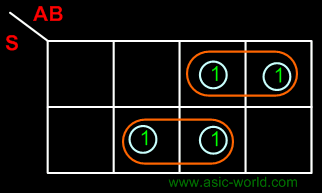Circuit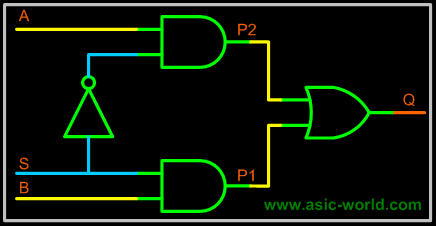Example : 4:1 MUX

A 4 to 1 line multiplexer is shown in figure below, each of 4 input lines I0 to I3 is applied to one input of an AND gate. Selection lines S0 and S1 are decoded to select a particular AND gate. The truth table for the 4:1 mux is given in the table below.Symbol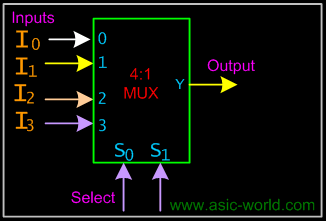Truth Table

 S1 S0 Y 0 0 I0 0 1 I1 1 0 I2 1 1 I3Circuit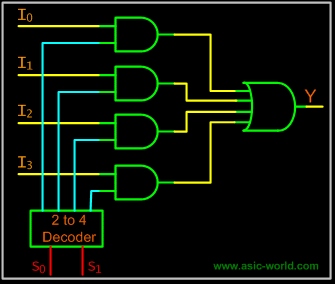Larger Multiplexers

Larger multiplexers can be constructed from smaller ones. An 8-to-1 multiplexer can be constructed from smaller multiplexers as shown below.Example - 8-to-1 multiplexer from Smaller MUXTruth Table

 S2 S1 S0 F 0 0 0 I0 0 0 1 I1 0 1 0 I2 0 1 1 I3 1 0 0 I4 1 0 1 I5 1 1 0 I6 1 1 1 I7Circuit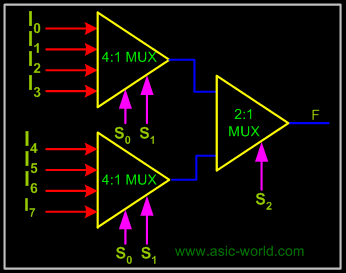Example - 16-to-1 multiplexer from 4:1 mux